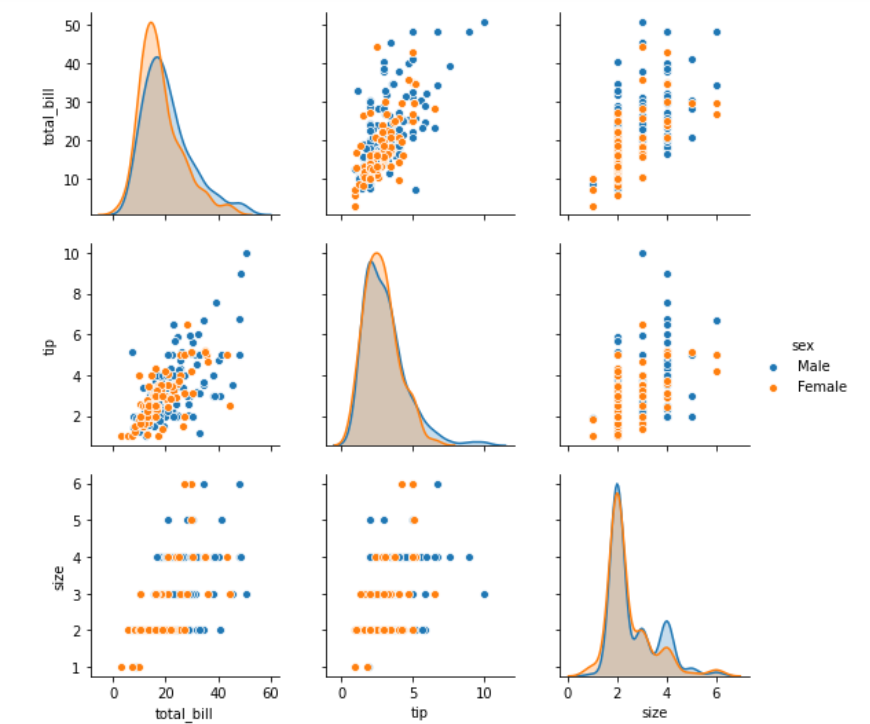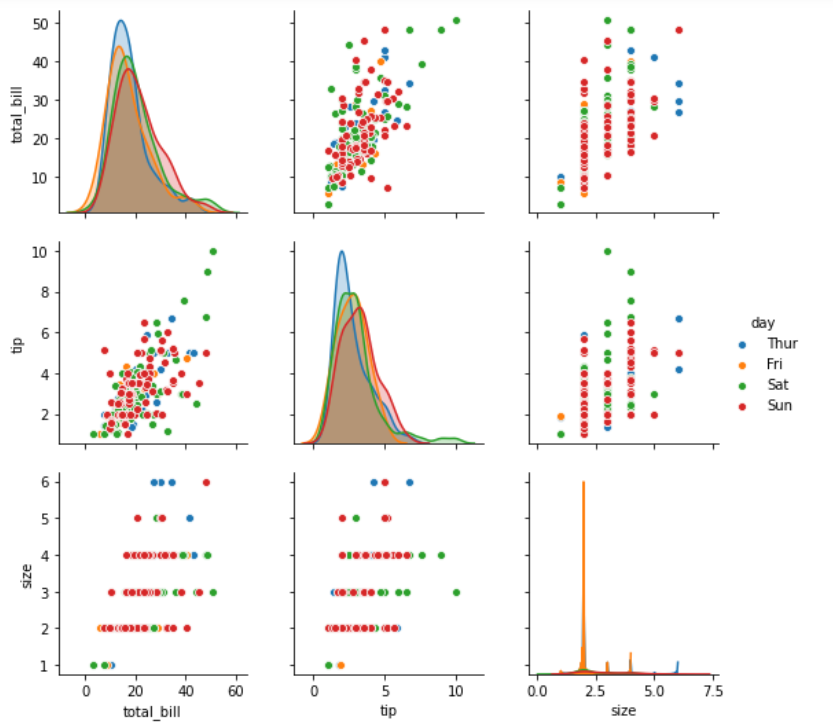# Python – seaborn.pairplot() method

• Last Updated : 15 Jul, 2020

#### Prerequisite: Seaborn Programming Basics

Seaborn is a Python data visualization library based on matplotlib. It provides a high-level interface for drawing attractive and informative statistical graphics. Seaborn helps resolve the two major problems faced by Matplotlib; the problems are ?

• Default Matplotlib parameters
• Working with data frames

As Seaborn compliments and extends Matplotlib, the learning curve is quite gradual. If you know Matplotlib, you are already half way through Seaborn.

Attention geek! Strengthen your foundations with the Python Programming Foundation Course and learn the basics.

To begin with, your interview preparations Enhance your Data Structures concepts with the Python DS Course. And to begin with your Machine Learning Journey, join the Machine Learning - Basic Level Course

### seaborn.pairplot() :

To plot multiple pairwise bivariate distributions in a dataset, you can use the pairplot() function. This shows the relationship for (n, 2) combination of variable in a DataFrame as a matrix of plots and the diagonal plots are the univariate plots.

```                        seaborn.pairplot( data, \*\*kwargs )

```

Seaborn.pairplot uses many arguments as input, main of which are described below in form of table:

Below is the implementation of above method:

## Python3

 `# importing packages``import` `seaborn``import` `matplotlib.pyplot as plt`` ` `############# Main Section ############``# loading dataset using seaborn``df ``=` `seaborn.load_dataset(``'tips'``)``# pairplot with hue sex``seaborn.pairplot(df, hue ``=``'sex'``)``# to show``plt.show()`` ` `# This code is contributed by Deepanshu Rustagi.`

#### Output :## Python3

 `# importing packages``import` `seaborn``import` `matplotlib.pyplot as plt`` ` `############# Main Section ############``# loading dataset using seaborn``df ``=` `seaborn.load_dataset(``'tips'``)``# pairplot with hue day``seaborn.pairplot(df, hue ``=``'day'``)``# to show``plt.show()`` ` `# This code is contributed by Deepanshu Rustagi.`

#### Output :My Personal Notes arrow_drop_up